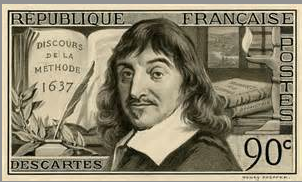newcommand("\pll","\u2227")
newcommand("\and","\u002e\u2227\u002e")
newcommand("\or","\u2228")

Analytic Geometry, Summary and Preview

\textit{$\C$ 2010, 2011, 2015 Prof. George K. Francis, Mathematics Department,
University of Illinois}

\begin{document}
16feb15
\maketitle\section{Introduction}
The Renaissance gave birth to the most profound innovation since Euclid,
the familiar analytic geometry you learned in high school. Envisioned by
Ren\'e Descartes(1595-1650) and realized by Pierre de Fermat (1607-1665),
it moved the basis of geometry from intuitively obvious postulates to the
universally recognized properties of numbers. It also replaced Euclid's
method of deducing all theorems from the postulates (Axiomatic Method) to
the algebraic reasoning and calculation (Analytic Mathod).

In this section of the course we will address the following topics to assist
our crossing the bridge from Euclidean to non-Euclidean (hyperbolic) geometry.
\begin{itemize}
\item Cartesian geometry is a model of the axiomatic system of Euclidean geometry bases Birkhoff's four axioms.
\item In particular, the fundamental role that similarity plays in nailing
the essential difference in the two geometries.
\item Contemporary forms of analytic geometry, namely vectors and functions
of complex numbers.
\end{itemize}
\section{Birkhoff's Axioms}
As we have seen, Euclid's postulate are not adequate to serve as axioms for
this geometry. He omitted certain axioms on continuity, plane separation,
and congruence, although he clearly assumed and used them in his proofs.
Hilbert's complete set of axioms are, however, too numerious and technical
for our course. Instead we will use Birkhoff's shorter list of only four
axioms.

Since we want to review analytic geometry anyway, but never review without
introducing challenging new ideas, we will show how analytic geometry is
a model of an axiomatic system based on Birkhoff's axioms. This will also
give you an idea what we plan to do for non-Euclidean geometry. There we
we will build several models of non-Euclidean geomtry inside the Cartesian
plane.

For now, refer to the second half of
Axiomatic Systems in Geometry section 6.

\section{Similarity, AAA, and symSAS}
The first of Birkhoff's axioms is just Euclid's postulate that two points
determine a unique line. The next two, the \textit{Ruler and Protractor
Axioms} establish the connection between geometry and algebra. It is the
fourth axiom,
symSAS  which requires
and deserves some explanation.

\end{document} 
~